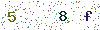﻿ LEDs Test Method: Basic Radiation Quantity and Photometric Quantity - LISUNLISUN

# LEDs Test Method: Basic Radiation Quantity and Photometric Quantity

Launching, transmitting and receiving the energy in the form of radiation, the unit is J (joule).

Radiation quantity is usually called radiant power Pe, it is the power launched, transmitted and received by the radiation source. The unit is watts (W), t is time, and the radiation quantity can be defined as

Φe =dQe/dt

Radiation intensity is defined as the ratio of radiant flux dΦe and the solid angle dΩ which is in a given direction inside, away from the point radiation source (or radiation source surface). The unit is watts/steradian (W/sr).

Ie =dΦe/dΩThe ratio of the radiation intensity dIe of one point on the radiation source area dS along a given direction and the frontal project area perpendicular to a given direction is defined as the radiation brightness. The unit is W/(sr·m2)The ratio of the radiation flux dΦe of one point exposed on the radiation receiving area and the point area dA, namely Ee=dΦe/dA, the unit is W/m2.

2. Photometric Quantity

2.1 Quantity of Light or Luminance Energy

It refers to the product of the flux and the light irradiation time, the unit is lumen seconds.

2.2 Φv Luminous Flux

It refers to the quantity of light emitted by the light source in unit of time, the unit is lumen, and symbol is lm.

Φv=dQv/dt

2.3 Iv Luminous Intensity

It refers to the ratio of the luminous flux dΦv contained in a small solid angle in a given derection and the solid angle dΩ. The unit is candela (cd).

Iv=dΦv /dΩ

2.4 Lv Luminance ( Luminance Meter )

It refers to the ratio of the luminous intensity dIv of one point area on the light source surface in a given direction and the frontal projected area perpendicular to the plane in a given direction. The unit is cd/mz.

Lv=dIv /(dScosθ)=d2(dΩdScosθ)

2.5 Ev Illuminance

It refers to the ratio of the luminous flux dΦv of the area exposed on the light receiving surface and the area dS. The unit is lux (lx).

Ev=dΦv /dS